# Main idea

The authors observe that commonly used activation functions are

• a. not learnable
• b. do not regard spatial relationships.

Hypothesising that a more sophisticated activation function addressing observations a. and b. could enable a CNN to make more efficient use of its parameters, they show how using their proposed activation function, called xUnit,

• 1. leads to reduced number of overall network parameters (less network depth)
• 2. optimises the ratio of parameters used for convolution and activation.

# A new learnable spatial activation function: xUnit

As shown in the figure below, the activation function can be interpreted as a transform of the input signal $$z_k$$ resulting in a scalar output which is then multiplied with $$z_k$$ to obtain the layer output $$x_{k+1}$$. The idea of xUnit is to use a transform which is learnable and which

• introduces a non-linearity (ReLU)
• regards spatial context (convolution)
• features gating map in $$[0,1]$$ (gaussian).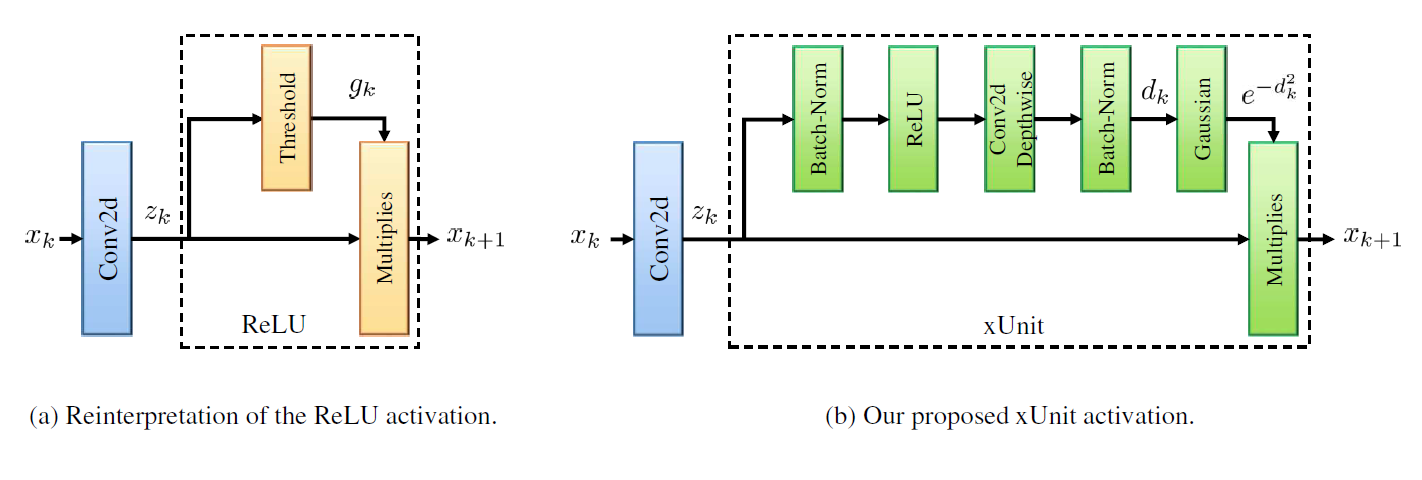# Experiments

Since the xUnit is introducing a significant amount of additional parameters the main question is if the use of this activation function improves the tradeoff between number of network parameters and network performance.

#### Choice of xUnit hyperparams

The figure below shows the comparison of a CNN architecture consisting of ‘Conv-BN-ReLU’ layers with its xUnit-augmented version in which layers are discarded to match the same number of parameters, on a denoising task (BSD dataset, 400 images). All xUnit configurations outperform the basic CNN network.

The authors find a convolution filter size of $$9\times9$$ in the xUnits to be optimal.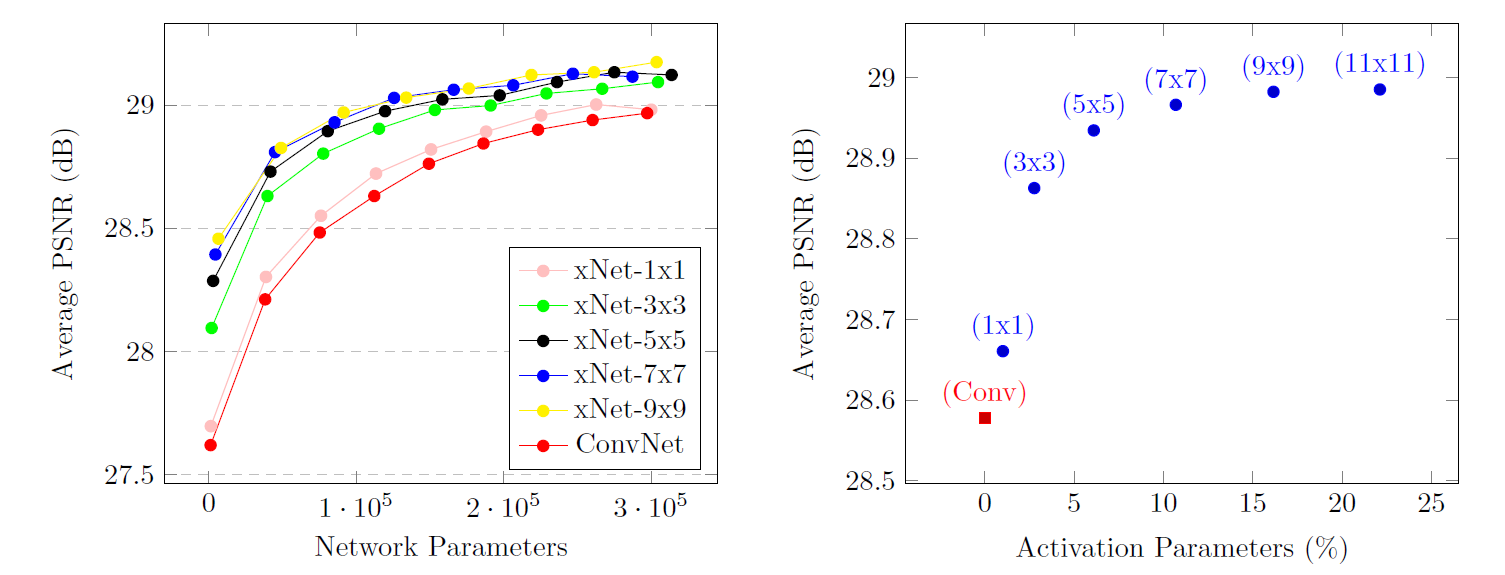Comparing the activation maps in a specific layer, it becomes apparent that the xUnit results in more dense activations and thus more feature maps contribute to the final result, as compared to the basic CNN approach where activations are sparse and mostly binary.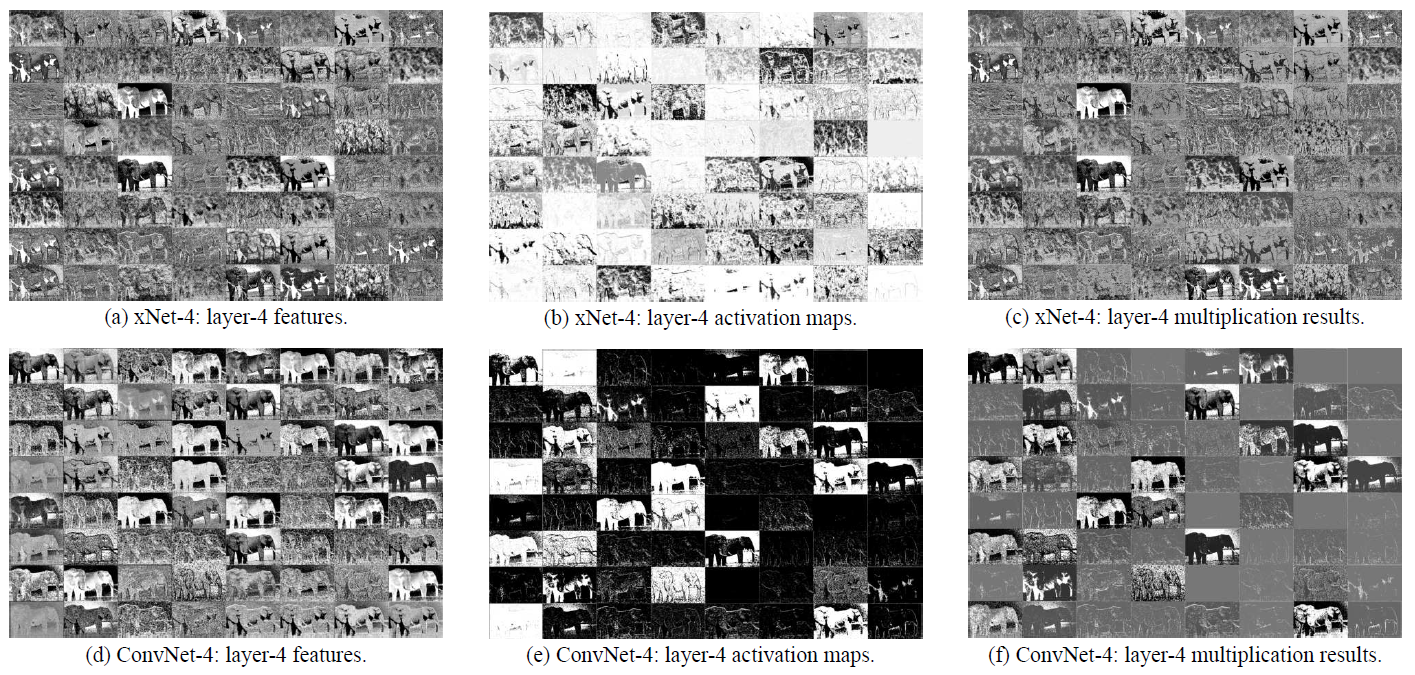#### Image denoising

The authors create their experiment dataset by adding gaussian noise to the images of the Berkely segmentation dataset (BSD, 400 images). They compare the performance to state of the art (non-NN) methods as well as the DnCNN (Denoise CNN). The latter serves as a basis for modification with their xUnit activation function and subsequent drop of network layers.

As can be seen in the table below, state of the art performance is achieved while significantly reducing the number of network parameters.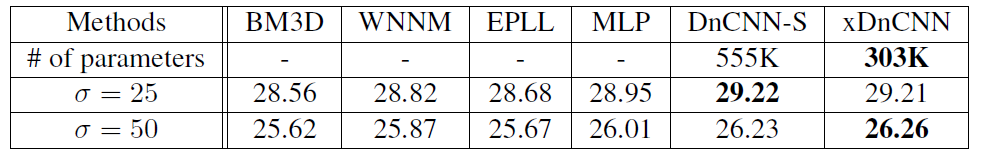Investigating the obtained image results, the output of their method seems to be slightly more appealing which might be due to the reported difference in activation maps (see above) potentially allowing for more complex aggregations of the feature maps.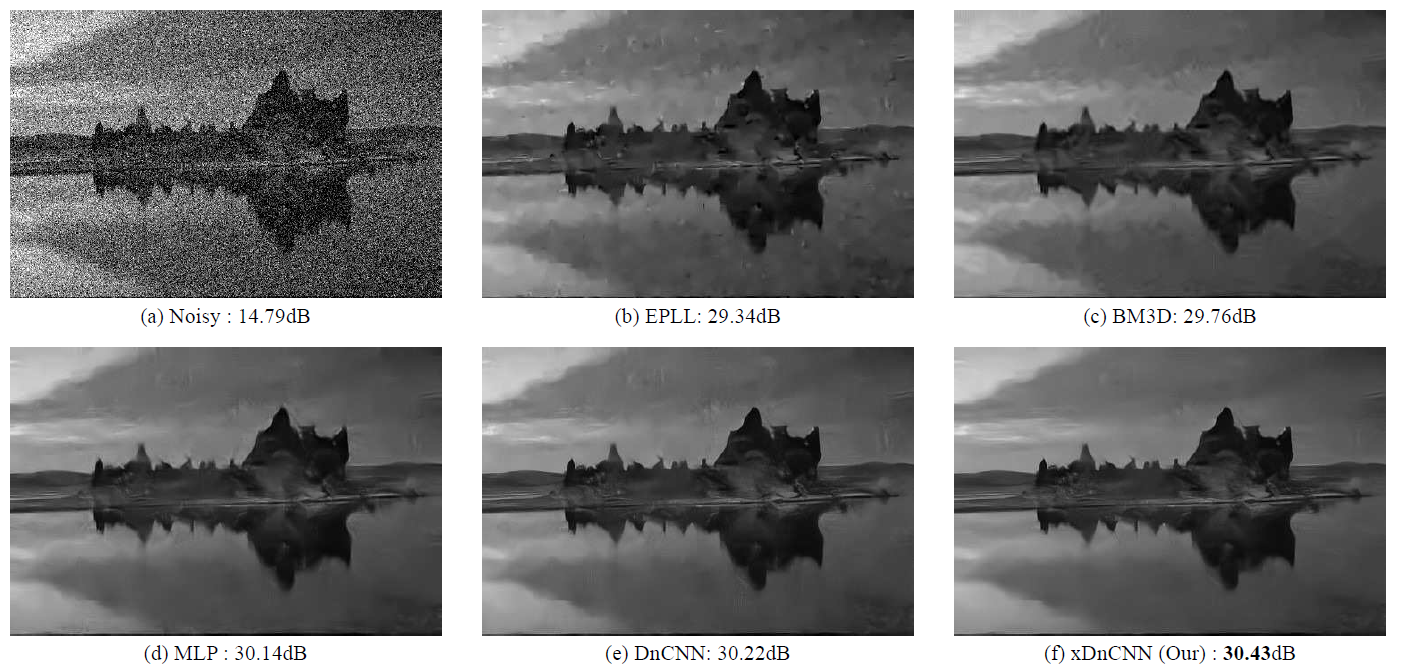#### Other experiments

In the same spirit as the denoising experiment, they show the efficiency of their proposal in the tasks of ‘de-raining’ as well as ‘super resolution’. Check the paper for more details.